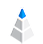# Mean vs. Median

The term “average” can often mislead us and hide valuable and reliable information form our data. It is hard to decide which number we need to use. In everyday statistics and news or even in scientific research, the result is often presented as the mean value. The median, however, gives you much better and more reliable information about your database, so we recommend using the median instead of the mean.

## The Difference Between Mean and Median

The mean is the average you are used to: add up all the numbers and then divide by the number of numbers. The median is the middle value in a list of numbers. To find the median, you need to list the numbers in numerical order, so you may have to rewrite your list first. Source of definitions

To see the difference, here is an example made from our own dataset, to illustrate the mean and median.

Click on the picture to enlarge.On the first chart, you can that see the income of companies in the middle range (25.56 to 1213). It is necessary to see this range because, in our dataset, some outlier values distort our results and visualization, but to see the main difference between median and mean, this chart is perfect. The green line shows the median (\$157K) and the red line shows the mean (\$603K). So in our case, we wanted to find our customers’ average income, and here is where the problem lies. The mean and the median show us very different information. So which do we trust? We recommend you to choose the median instead of the mean. Below you can read the reasons.

## The Outlier Problem

On the second chart, you can see the same dataset but visualized in full range (7.3 to 27302.93). That is a big range. It shows the income inequality too, but that is another topic.

Click on the picture to enlargeIt is also easy to see the difference between the median and the mean, but now we can clearly see how far the outlier value is.

## The Changing Mean

We can ask the question, What if we do not involve the outlier? Let’s see what happens when we exclude more and more outlier values.

Click on the picture to enlargeThe value of the mean will change (decrease), but the median will not until a bigger change occurs. Therefore, the median is a more reliable and more stable number than the mean. The outlier value will not throw off the result.

## Standard Deviation

Standard deviation is often used to support the understanding of the average. It helps to describe our results by not using one number only, but it is not understandable to everybody. Instead, it is better to use the midrange. For this, we can use the IQR (interquartile range), which can show us the range of the middle 50% of the values. However, what about the other 50%? It is better if we check the 10-90 percentile range- the middle 80% of the values. With this number, we can describe the bottom and the top 10% of our data.

## Histogram

It has been proven that people can understand shapes and visualized data better than just plain numbers. Therefore, we recommend using histograms. Below you can see one more reason to use it.You may have a big amount of minimum and maximum values but just a few from the middle. In our case, the mean and the median will be the same number (5), and without a histogram, you will not be able to see the real meaning of your data.

## Summary:

• Using the mean value can often mislead you and hide the truth
• Outliers can destroy the results, and the mean value will show a false picture of your data
• Visualize your data (graphs, charts, histogram, etc)
• Always know the background

Interesting Fact: The Friendship paradox states that on average, your friends have more friends than you, on average your friends sleep, drink and work more than you, and so on. The mean does not show the truth.

So use median and be a real data scientist!

Connect your data and start the exploration